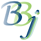# BBjSysGui::getMeasures

## Description

In BBj 13.0 and higher, this method returns the widths in unscaled pixels of the specified strings in the current active or focused window.

## Syntax

Return Value

Method

BBjVector

getMeasures(BBVector vector)

## Parameters

Parameter

Description

vector

BBjVector containing strings to be measured.

## Return Value

Returns the widths in unscaled pixels of the specified strings in the current active window as a BBjVector of integers. Throws an error if there is no current window.

## Remarks

This method is most useful in a distributed environment to reduce the number of blocking client/server requests.

## Example

Copy

BBjSysGui::getMeasures Example

``sysgui = untopen (sysgui)"X0"sysgui! = BBJAPI().getSysGui()window! = sysgui!.addWindow(50,50,600,200,"getMeasures",\$00090003\$)window!.setCallback(window!.ON_CLOSE,"eoj")font! = sysgui!.makeFont("Courier New",16,0)window!.setFont(font!)vector! = bbjapi().makeVector()vector!.add("The ")vector!.add("quick ")vector!.add("brown ")vector!.add("fox ")vector!.add("jumps ")vector!.add("over ")vector!.add("the ")vector!.add("lazy ")vector!.add("dog.")measures! = sysgui!.getMeasures(vector!)msg\$ = "Strings: " + str(vector!) + \$0a\$msg\$ = msg\$ + "Font: " + str(font!) + \$0a\$msg\$ = msg\$ + "Measures: " + str(measures!)print msg\$x = 10y = 100for i = 0 to vector!.size() - 1   print (sysgui)'textcolor'(rnd(255),rnd(255),rnd(255))   print (sysgui)'plottext'(x,y,vector!.get(i))   x = x + measures!.get(i)next ii = msgbox(msg\$,0,"getMeasures")process_eventseoj:release``